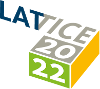#The 39th International Symposium on Lattice Field Theory (Lattice 2022)

Aug 8 – 13, 2022
Hörsaalzentrum Poppelsdorf
Europe/Berlin timezone

## Measurement of hadron masses in 2-color finite density QCD

Aug 11, 2022, 12:30 PM
20m
CP1-HSZ/0.011 (CP1-HSZ) - HS1 (CP1-HSZ)

### CP1-HSZ/0.011 (CP1-HSZ) - HS1

#### CP1-HSZ

450
Show room on map
Oral Presentation QCD at Non-zero Density

### Speaker

Kotaro Murakami (Yukawa Institute for Theoretical Physics, Kyoto University)

### Description

We investigate hadron masses in two-color QCD with $N_f=2$ at low temperature and finite density using lattice simulation. We calculate $\pi$ and $\rho$ meson masses and find the flipping of the spectral ordering of these two mesons near the transition between the hadronic and superfluid phases.
Furthermore, we measure hadron masses with isospin $I=0$ and $J^P = 0^{\pm}$. According to the analysis of the linear sigma model of finite-density two-color QCD with a diquark gap, the meson, diquark, and antidiquark states in such channels are mixed due to the $U(1)_B$ symmetry breaking in the superfluid phase. Our lattice study provides the two-point functions, which are consistent with the above prediction.

### Primary authors

Dr Daiki Suenaga (Strangeness Nuclear Physics Laboratory, RIKEN Nishina Center) Kotaro Murakami (Yukawa Institute for Theoretical Physics, Kyoto University) Etsuko Itou (RIKEN) Kei Iida (Department of Mathematics and Physics, Kochi University)